2021-09-21 10:03

# 这几个题，Python求解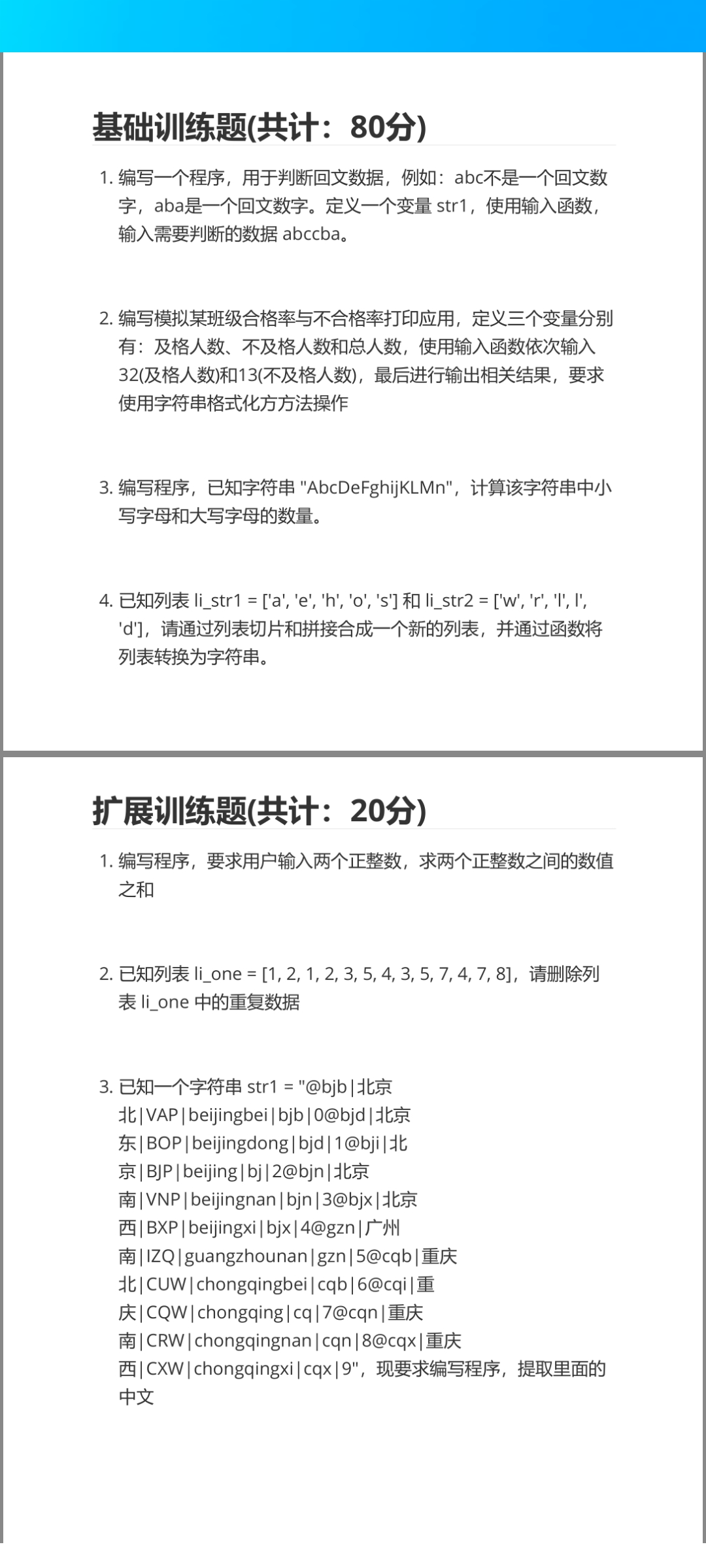• 收藏

#### 1条回答默认 最新

•Python GUI 2021-09-21 16:13
已采纳

直接上代码：

``````## 1.
checkstr = input("请输入需要判断的数据：")
if len(checkstr) != 1 and len(checkstr) % 2 != 0: #如果不是1或者不是双数
print("这不是回文数据！")
elif len(checkstr) == 1:
print("这是回文数据")
else:
length = len(checkstr)
one = checkstr[0:int((length / 2) + 1)] #把文本平均分成两部分，这是第一部分
two = checkstr[::-1][0:int((length / 2) + 1)] #这是第二部分，并且倒转了一下
if one == two: #如果两部分完全一样
print("这是回文数据")
else:
print("这不是回文数据！")
``````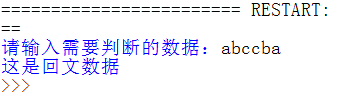``````## 2.
passnum = int(input("及格人数："))
unpassnum = int(input("不及格人数："))
allnum = passnum + unpassnum #总人数计算
print(passnum, unpassnum, allnum) #打印及格，不及格，一共人数
``````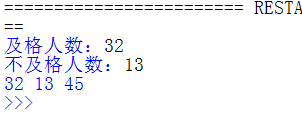``````## 3.
from string import ascii_lowercase
it = "AbcDeFghijKLMn"
lowernum = 0
uppernum = 0
for each in it:
if each in ascii_lowercase: #如果它在小写字母表里
lowernum += 1
else:
uppernum += 1
print("大写字母数量：" + str(uppernum))
print("小写字母数量：" + str(lowernum))
``````

Output(输出内容)
大写字母数量：6
小写字母数量：8

``````## 4.
li_str1 = ['a','e','h','o','s']
li_str2 = ['w','r','l','l','d'] #注：我实在看不清题目中那两个竖线是什么字符，就写了两个l
newlist = li_str1 + li_str2 #列表拼接
str_newlist = str(newlist) #转换成字符串
#如果题目的意思是将列表内容拼接起来而非单纯的转换，则改成这样
#str_newlist = "".join(newlist)
``````

下面是拓展题的答案

``````## 拓展1
a_ = abs(int(input("请输入正整数1："))) #为了保证内容一定是正整数，这里用了abs求绝对值
b_ = abs(int(input("请输入正整数2：")))
get = 0
if a_ != b_:
a = min([a_, b_])
b = max([a_, b_])
aon = a
while True:
aon += 1
if aon == b:
break
get += aon #将值相加
print("两个正整数之间的数值之和是：" + str(get))
#这里求得的值并不包括两个正整数本身，如果需要加上的话改成下面的代码
#print("两个正整数之间的数值之和是：" + str(get + a + b))
``````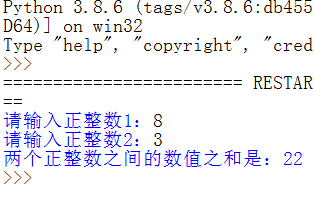``````## 拓展2
li_one = [1,2,1,2,3,5,4,3,5,7,4,7,8]
li_one = list(set(li_one)) #集合里面是不会有重复数据的
``````
``````## 拓展3
from string import printable
str1 = "床前chuangqian#\$%@明月光"
#那一串内容太长了，所以我就省略了部分，但效果是一样的，都适用
get = ""
for each in str1:
if each not in printable: #如果是中文
get += each
print("提取出中文：")
print(get)
``````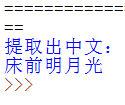这些就是我编写的以上问题的全部答案，有帮助的话麻烦给个采纳！

已采纳该答案
1 打赏 评论